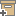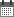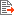ניהול
קהילה:
אסיף מאגר המחקר החקלאי
A note on the effective parameters of the convection-dispersion equationBack to searchPrevious item
Next item
Year:
2002
Source of publication :
Water Resources Research
Authors :
רוסו, דוד
;
.
Volume :
38
Co-Authors:
Russo, D., Department of Environmental Physics, I. I. S. W./E. S., Volcani Center, Bet Dagan 50250, Israel
Facilitators :
From page:
91
To page:
97
(
Total pages:
7
)
Abstract:
We focus on the analysis of the effective parameters of the one-dimensional convection-dispersion equation (CDE), estimated from the depth distribution of the solute concentration at a given observation time. This situation should be distinguished from that in which the effective parameters of the one-dimensional CDE are estimated from the temporal distribution of the solute concentration at a given arbitrary soil depth. In this latter case, both the mean and the variance of the solute travel time probability density function increase linearly with travel distance, and the resultant effective solute velocity, Ve, and dispersion coefficient, De, are constant, independent of travel distance. Results of the present analysis suggest that under both first-type and third-type upper boundary conditions, the resultant Ve and De are time dependent. Consequently, the effective dispersivity, λe = De/Ve, increases with travel time or distance as the solute body first invades the flow system and tends to approach a constant value (i.e., Fickian behavior) after the solute body has traveled a distance of few tens of pore-scale dispersivity. These findings, which stem from the Lagrangian nature of the dispersion phenomenon, are consistent with the results of first-order analyses in saturated and partially saturated heterogeneous formations, based on the theory of dispersion by continuous motions. The findings presented in this technical note have important implications regarding the estimation of parameters of the one-dimensional CDE from the depth distribution of the solute concentration at a given observation time, and the subsequent prediction of solute movement.
Note:
Related Files :
Boundary conditions
convection
Heat convection
probability density function
soil
Soils
Solutes
Solute transport
עוד תגיות
תוכן קשור
More details
DOI :
Article number:
Affiliations:
Database:
סקופוס
Publication Type:
מאמר
;
.
Language:
אנגלית
Editors' remarks:
ID:
29194
Last updated date:
02/03/2022 17:27
Creation date:
17/04/2018 00:45Scientific Publication
A note on the effective parameters of the convection-dispersion equation
38
Russo, D., Department of Environmental Physics, I. I. S. W./E. S., Volcani Center, Bet Dagan 50250, Israel
A note on the effective parameters of the convection-dispersion equation
We focus on the analysis of the effective parameters of the one-dimensional convection-dispersion equation (CDE), estimated from the depth distribution of the solute concentration at a given observation time. This situation should be distinguished from that in which the effective parameters of the one-dimensional CDE are estimated from the temporal distribution of the solute concentration at a given arbitrary soil depth. In this latter case, both the mean and the variance of the solute travel time probability density function increase linearly with travel distance, and the resultant effective solute velocity, Ve, and dispersion coefficient, De, are constant, independent of travel distance. Results of the present analysis suggest that under both first-type and third-type upper boundary conditions, the resultant Ve and De are time dependent. Consequently, the effective dispersivity, λe = De/Ve, increases with travel time or distance as the solute body first invades the flow system and tends to approach a constant value (i.e., Fickian behavior) after the solute body has traveled a distance of few tens of pore-scale dispersivity. These findings, which stem from the Lagrangian nature of the dispersion phenomenon, are consistent with the results of first-order analyses in saturated and partially saturated heterogeneous formations, based on the theory of dispersion by continuous motions. The findings presented in this technical note have important implications regarding the estimation of parameters of the one-dimensional CDE from the depth distribution of the solute concentration at a given observation time, and the subsequent prediction of solute movement.
Scientific Publication
You may also be interested in